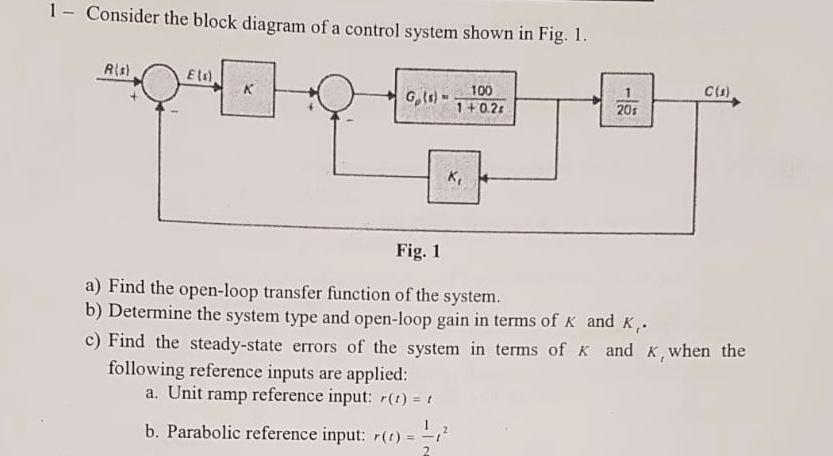# 1- Consider the block diagram of a control system shown in Fig. 1 Rts) E ts) C(s) Gt-11027 20s Fi...

###### Question:1- Consider the block diagram of a control system shown in Fig. 1 Rts) E ts) C(s) Gt-11027 20s Fig. 1 a) Find the open-loop transfer function of the system. b) Determine the system type and open-loop gain in terms of K and K, c) Find the steady-state errors of the system in terms of K and K,when the following reference inputs are applied: a. Unit ramp reference input: ) b. Parabolic reference input: r()